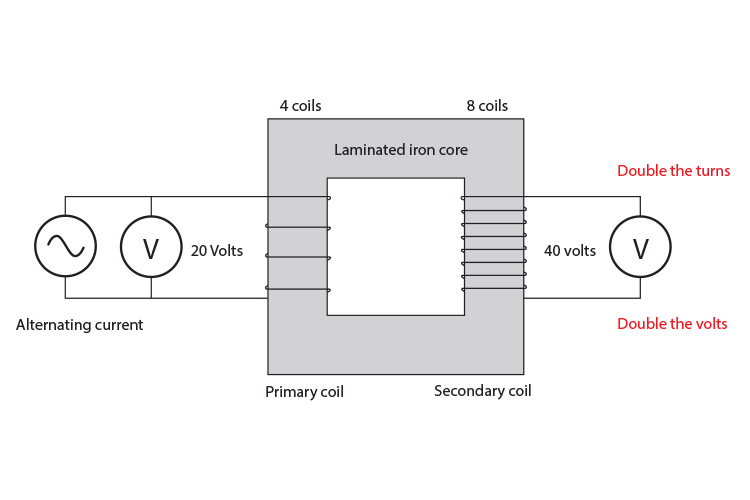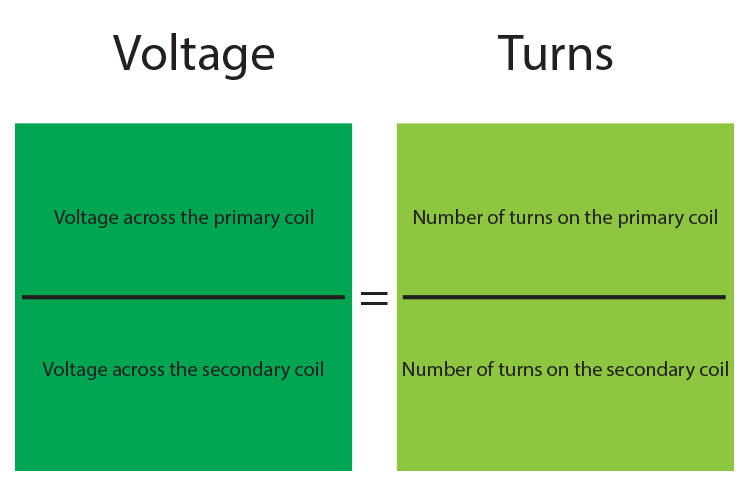# Transformer – changing the number of turns

Scientists then changed the number of coils on the primary coil and secondary coil. They found that if you have twice as many turns on the secondary coil then twice the voltage will be present on the secondary coil.Number turns xx2 = Voltage xx2 and Number turns xx1/2 = Voltage xx1/2

Or in terms of formula:(NOTE: the two different coloured shades will help you remember voltage is one side and turns are the other (colours can be a great mnemonic))

or

V_1/V_2=N_1/N_2